• 斯坦福课程实验。包含具体要求及代码实现，可直接运行
• 机器学习实验一线性回归 实验题目 1 、 一元线性回归 题目： 应用一元线性回归预测移动餐车的利润。假设你是一家餐饮连锁店的CEO， 考虑在不同的城市开辟新店。该餐饮店已在许多城市拥有移动餐车，现有各个城 市移动...
机器学习实验一线性回归
实验题目
1 、 一元线性回归 题目：     应用一元线性回归预测移动餐车的利润。假设你是一家餐饮连锁店的CEO， 考虑在不同的城市开辟新店。该餐饮店已在许多城市拥有移动餐车，现有各个城 市移动餐车的利润和城市人口的数据。这些数据将帮助你选择在哪个城市进行新 店扩张。请按要求完成实验。
数据集：     文件ex1data1.txt 为该实验部分的数据集，第一列表示城市人口（单位为万 人），第二列表示该城市的移动餐车的利润（单位为万美元，若利润为负值，表 示损失）。
步骤与要求： 1）在开始任务之前，进行数据的可视化对于了解数据特征是很有帮助的。 请你导入数据并以人口为横坐标，利润为纵坐标画出散点图并观察数据分布特征。 （建议：用python 的matplotlib） 2）将线性回归参数初始化为0，然后计算代价函数（cost function）并求出 初始值。 3）使用线性回归的方法对数据集进行拟合，并用梯度下降法求解线性回归 参数。（eg：迭代次数=1500，alpha=0.01） 4）画出数据的拟合图形。 5）预测人口数量为35000 和70000 时，利润为多少。
2 、 多元线性回归 题目：     应用多元线性回归预测房价。假设你打算出售你的房子，你想知道房子的市 场价应该设为多少比较合适。一种方法就是收集最近的房屋销售信息并设计一个 房屋价格模型。请按要求完成实验。
数据集：     文件ex1data2.txt 为该实验部分的数据集，第一列表示房屋的面积（平方英 尺），第二列表示房间数目，第三列表示房屋价格。
步骤与要求： 1）导入数据，通过观察，容易发现房屋面积的大小约是房间数量的1000 倍。 当特征数量级不同时，对进行特征缩放能够使梯度下降更快地收敛。请对这两个 特征进行归一化处理。 2）使用梯度下降法求解线性回归参数。尝试使用不同的alpha（学习率）进 行实验，找到一个合适的alpha 使算法快速收敛。思考alpha 的大小对于算法性 能的影响。 3）使用你认为最佳的alpha 运行梯度下降法求出线性回归参数，然后预测房 屋面积为 1650 平方英尺，房间数量为 3 时，房屋的价格。
题目一
import numpy as np
import matplotlib.pyplot as plt
#设置字体
plt.rcParams['font.sans-serif'] =['SimHei']

def LoadFile(filename):
data = np.loadtxt(filename, delimiter=',', unpack=True, usecols=(0, 1))
x = np.transpose(np.array(data))
y = np.transpose(np.array(data))
return x, y

#加载样本数据

if __name__ == '__main__':
x, y = LoadFile('ex1data1.txt')
learn_rate=0.01  #设置超参数,学习率
iter=1500    #迭代次数
display_step=50  #每50次迭代显示一下效果

#初始化为0
w=0
b=0

#训练模型
mse=[] #存放每次迭代的损失值
for i in range(0,iter+1):
#求偏导
dL_dw=np.mean(x*(w*x+b-y))
dL_db=np.mean(w*x+b-y)
#更新模型参数
w=w-learn_rate*dL_dw
b=b-learn_rate*dL_db
#得到估计值
pred=w*x+b
#计算损失(均方误差)
Loss=np.mean(np.square(y-pred))/2
mse.append(Loss)
if i%display_step==0:
print("i:%i,Loss:%f,w:%f,b:%f"%(i,mse[i],w,b))

print("城市人口为35000时的预测餐车利润:%f"%(3.5*w+b))
print("城市人口为70000时的预测餐车利润:%f"%(7*w+b))
#模型和数据可视化
plt.figure(figsize=(20,4))
plt.subplot(1,3,1)
#绘制散点图
#张量和数组都可以作为散点函数的输入提供点坐标
plt.scatter(x,y,color="red",label="数据集")
plt.scatter(x,pred,color="green",label="梯度下降法")
plt.plot(x,pred,color="blue")

#设置坐标轴的标签文字和字号
plt.xlabel("城市人口（万人）",fontsize=14)
plt.ylabel("餐车利润（万美元）",fontsize=14)

#在左上方显示图例
plt.legend(loc="upper left")

#损失变化可视化
plt.subplot(1,3,2)
plt.plot(mse)
plt.xlabel("迭代次数",fontsize=14)
plt.ylabel("损失值",fontsize=14)
#估计值与标签值比较可视化
plt.subplot(1,3,3)
plt.plot(y,color="red",marker="o",label="数据集")
plt.plot(pred,color="blue",marker="o",label="预测利润")
plt.legend()
plt.xlabel("sample",fontsize=14)
plt.ylabel("price",fontsize=14)
#显示整个绘图
plt.show()


题目二
import numpy as np
import matplotlib.pyplot as plt
from mpl_toolkits.mplot3d import Axes3D

def LoadFile(filename):
data = np.loadtxt(filename, delimiter=',', unpack=True, usecols=(0, 1, 2))
x = np.transpose(np.array(data))
y = np.transpose(np.array(data))
z = np.transpose(np.array(data))
return x, y, z

if __name__ == '__main__':
area, room, price = LoadFile('ex1data2.txt')
num=len(area) #样本数量
x0=np.ones(num)
#归一化处理，这里使用线性归一化
x1=(area-np.average(area))/(area.max()-area.min())
x2=(room-np.average(room))/(room.max()-room.min())
#堆叠属性数组，构造属性矩阵
#从(16,)到(16,3),因为新出现的轴是第二个轴所以axis为1
X=np.stack((x0,x1,x2),axis=1)
#print(X)
#得到形状为一列的数组
Y=price.reshape(-1,1)
#print(Y)
learn_rate=0.001    #设置超参数
iter=1500   #迭代次数
display_step=50    #每50次迭代显示一下效果

#设置模型参数初始值
W=[,
,
]
#训练模型
mse=[]
for i in range(0,iter+1):
#求偏导
dL_dW=np.matmul(np.transpose(X),np.matmul(X,W)-Y)   #XT(XW-Y)
#更新模型参数
W=W-learn_rate*dL_dW
#得到估计值
PRED=np.matmul(X,W)
#计算损失(均方误差)
Loss=np.mean(np.square(Y-PRED))/2
mse.append(Loss)
if i % display_step==0:
print("i:%i,Loss:%f"%(i,mse[i]))
xx0=np.ones(1)
xx1=(1650.0-np.average(area))/(area.max()-area.min())
xx2=(3.0-np.average(room))/(room.max()-room.min())
XX=[xx0,xx1,xx2]
print("房屋面积为1650平方英尺房间数量为3时预测房屋的价格:%f"%(np.matmul(XX,W)))

#结果可视化
plt.rcParams['font.sans-serif'] =['SimHei']
plt.figure(figsize=(12,4))
#损失变化可视化
plt.subplot(1,2,1)
plt.plot(mse)
plt.xlabel("迭代次数",fontsize=14)
plt.ylabel("损失值",fontsize=14)
#估计值与标签值比较可视化
plt.subplot(1,2,2)
PRED=PRED.reshape(-1)
plt.plot(price,color="red",marker="o",label="数据集")
plt.plot(PRED,color="blue",marker="o",label="预测房价")
plt.xlabel("sample",fontsize=14)
plt.ylabel("price",fontsize=14)
plt.legend()
plt.show()

fig = plt.figure()
ax = Axes3D(fig)
ax.scatter(area, room, price, color="red")
ax.set_zlabel('price', fontdict={'size': 15, 'color': 'red'})
ax.set_ylabel('room', fontdict={'size': 15, 'color': 'red'})
ax.set_xlabel('area', fontdict={'size': 15, 'color': 'red'})
ax.scatter(area, room, PRED,color="b")
XX, YY = np.meshgrid(area, room)
ax.plot_surface(XX,
YY,
Z=W[:,0]*x0+W[:,0]*((XX-np.average(area))/(area.max()-area.min()))
+W[:,0]*((YY-np.average(room))/(room.max()-room.min())),
color='g',
alpha=0.9
)

plt.show()


注：细节方面大家自己看
实验工程链接实验工程链接


展开全文• 机器学习实验二——逻辑回归 实验报告 组员：张继伟 谢正宇 樊佳婷 孙晶铭 刘怡聪 题目 假设你是某大学招生主管，你想根据两次考试的结果决定每个申请者的录取机会。现有以往申请者的历 史数据，可以此作为训练集...
机器学习实验二——逻辑回归 实验报告
组员：张继伟 谢正宇 樊佳婷 孙晶铭 刘怡聪
题目
假设你是某大学招生主管，你想根据两次考试的结果决定每个申请者的录取机会。现有以往申请者的历 史数据，可以此作为训练集建立逻辑回归模型，并用其预测某学生能否被大学录取。请按要求完成实 验。建议使用 python 编程实现。 数据集
文件 ex2data1.txt 为该实验的数据集，第一列、第二列分别表示申请者两次考试的成绩，第三列表示录 取结果（1 表示录取，0 表示不录取）。
步骤与要求
1）请导入数据并进行数据可视化，观察数据分布特征。（建议用 python 的matplotlib） 2）将逻辑回归参数初始化为 0，然后计算代价函数（cost function）并求出初始值。 3）选择一种优化方法求解逻辑回归参数。 4）某学生两次考试成绩分别为 42、85，预测其被录取的概率。 5）画出分类边界。（选做）
实验过程与结果分析
# 导入Matploylib库
from matplotlib import pyplot as plt
from numpy import *
import matplotlib.ticker as ticker
import numpy as np
import scipy.optimize as opt

# 定义h(x)预测函数:theat为转置后的矩阵
def hypothesis(theta, x):
return np.dot(x, theta)

# 定义sigmoid函数
def sigmoid(theta, x):
z = hypothesis(theta, x)
return 1.0 / (1 + exp(-z))

# 定义代价函数
def cost(theta, X, y):
return np.mean(-y * np.log(sigmoid(theta, X)) - (1 - y) * np.log(1 - sigmoid(theta, X)))

# 梯度下降函数
def gradient(theta, X, y):
return (1 / len(X)) * X.T @ (sigmoid(theta, X) - y)

'''
绘图：绘制训练数据的散点图和h(x)预测函数对应的直线
'''
def draw():
# 定义x y数据 x1 y1:未通过 x2 y2:通过
x1 = []
y1 = []
x2 = []
y2 = []

# 导入训练数据
train_data = open("ex2data1.txt")
lines = train_data.readlines()
for line in lines:
scores = line.split(",")
# 去除标记后面的换行符
isQualified = scores.replace("\n", "")
# 根据标记将两次成绩放到对应的数组
if isQualified == "0":
x1.append(float(scores))
y1.append(float(scores))
else:
x2.append(float(scores))
y2.append(float(scores))

# 设置标题和横纵坐标的标注
plt.xlabel("Exam 1 score")
plt.ylabel("Exam 2 score")

# 设置通过测试和不通过测试数据的样式。其中x y为两次的成绩，marker:记号形状 color:颜色 s:点的大小 label:标注
plt.scatter(x1, y1, marker='o', color='red', s=15, label='Not admitted')
plt.scatter(x2, y2, marker='x', color='green', s=15, label='Admitted')

# 标注[即上两行中的label]的显示位置：右上角
plt.legend(loc='upper right')

# 设置坐标轴上刻度的精度为一位小数。因训练数据中的分数的小数点太多，若不限制坐标轴上刻度显示的精度，影响最终散点图的美观度
plt.gca().xaxis.set_major_formatter(ticker.FormatStrFormatter('%.1f'))
plt.gca().yaxis.set_major_formatter(ticker.FormatStrFormatter('%.1f'))

# 设置训练得到的模型对应的直线，即h(x)对应的直线
# 设置x的取值范围：[30, 110]步长为10
x = np.arange(30, 110, 10)
y = (-result.x - result.x * x) / result.x
predict=(-result.x-result.x*42)/result.x
print("当第一次考试成绩为42时，最小可被接收的第二次成绩为",predict)
if predict-85<0:
print("这个学生可以被接收！")
else:
print("这个学生不能被接收！")
plt.plot(x, y)

# 显示
plt.show()

'''
数据预先处理：将两次成绩与是否通过测试的标记分别生成矩阵，并将标记的矩阵转置。
'''
def init_data():
# 两次成绩对应的特征矩阵
data = []
# 标记对应的矩阵
label = []

# 读取文件
train_data = open("ex2data1.txt")
lines = train_data.readlines()
for line in lines:
scores = line.split(",")
# 去除标记后面的换行符
isQualified = scores.replace("\n", "")
# 添加特征x0，设置为1
data.append([1, float(scores), float(scores)])
label.append(int(isQualified))

# 标记矩阵转置，返回特征矩阵和标记矩阵
return np.array(data), np.array(label).transpose()

'''
主函数
'''
if __name__ == '__main__':
# 初始化数据
X, y = init_data()
# 初始化theta:三行一列的0矩阵
theta = np.zeros((3, 1))
# 使用minimize函数求解
result = opt.minimize(fun=cost, x0=theta, args=(X, y), method='Newton-CG', jac=gradient) #method求极值的方法 Newton-CG算法最小化一个或多个变量的标量函数。
#minimize函数参数详解:https://docs.scipy.org/doc/scipy/reference/generated/scipy.optimize.minimize.html#scipy.optimize.minimize
#print(result)
# 绘图
draw()


代码细节自己看
也可以下载实验报告
工程链接工程链接


展开全文• 实验要求 代码如下： import math import sys import numpy as np import pandas as pd # 读取数据 data = pd.read_csv('./train.csv', encoding='UTF8') # 排除前三列，并处理所有的NR值 data = data.iloc[:,...
实验要求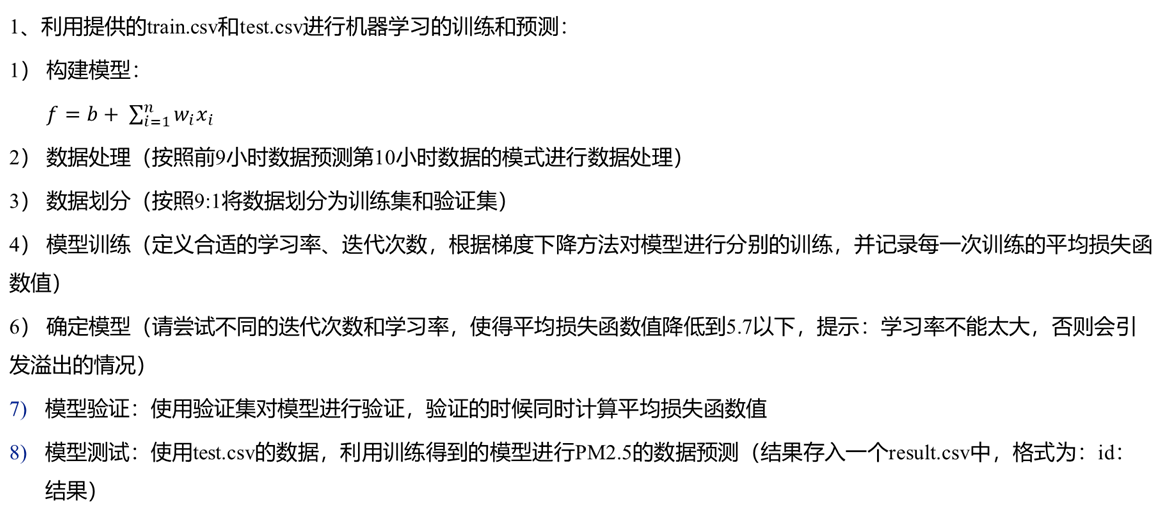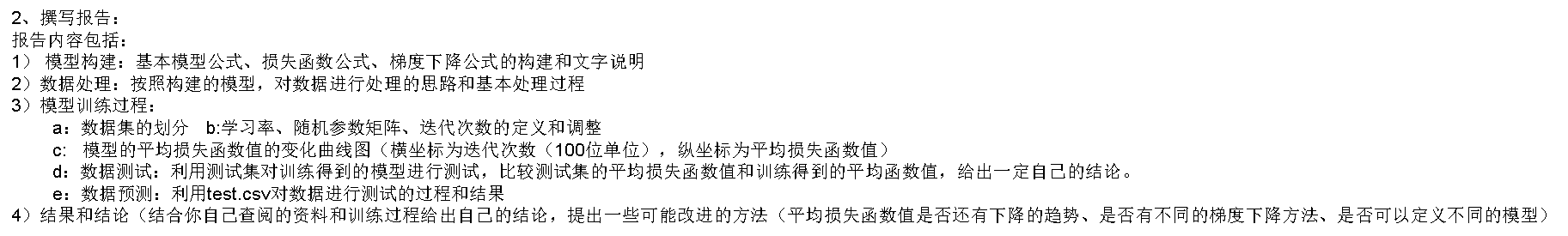代码如下：

import math
import sys

import numpy as np
import pandas as pd

# 读取数据
data = pd.read_csv('./train.csv', encoding='UTF8')

# 排除前三列，并处理所有的NR值
data = data.iloc[:, 3:]
data[data == 'NR'] = 0

# 将数据转换为数组
raw_train_data = data.to_numpy()

# 目前数据，每个月有20天的数据（1-20号），每天有18个参数，24组观测值（24小时）
# 即：如果按月计算，总共有12个月，每个月20天，需要的Shape为：18 * 480

month_data = {}
for month in range(12):
sample = np.empty([18, 480], dtype=float)
for day in range(20):
# 每天24组数据，则第i天为： i *24 : (i+1) * 24
# 其在原始数据的行范围为：month * 20 + day : (18 * (20 * month + day + 1)
sample[:18, day * 24: (day + 1) * 24] = raw_train_data[18 * (month * 20 + day): 18 * (20 * month + day + 1),
:24]
month_data[month + 1] = sample

# 由于数据需要转换为y = b + w1x1 + w2x2 + ... + w18*9 x18*9
# 对于每个月，每10个小时分成一组，由前9个小时的数据来预测第10个小时的PM2.5，把前9小时的数据（排除PM25）放入x
# 窗口的大小为10，从第1个小时开始向右滑动，每次滑动1小时。因此，每个月都有471组这样的数据。

x = np.empty([12 * 471, 18 * 9], dtype=float)
y = np.empty([12 * 471, 1], dtype=float)
total = 0
for month in range(12):  # 12个月
for day in range(20):  # 20天1个月
for hour in range(24):  # 1天24个小时
if (day * 24 + hour + 9) < 480:
x_temp_data = month_data[month + 1][:18, day * 24 + hour: day * 24 + hour + 9].reshape(1, 162)
y_temp_data = month_data[month + 1][9:10, day * 24 + hour + 9:day * 24 + hour + 10]
# print(month_data[month + 1][9:10, day * 24 + hour + 9:day * 24 + hour + 10])
x[total:total + 1] = x_temp_data
y[total:total + 1] = y_temp_data
total = total + 1

# 标准化，使用 x - u / delta
mean_x = np.mean(x, axis=0)  # 18 * 9
std_x = np.std(x, axis=0)

for i in range(len(x)):
for j in range(len(x)):
x[i][j] = (x[i][j] - mean_x[j]) / std_x[j]

# 为x多添加一列，全为1，表示*b的结果
x = np.concatenate((x, np.ones([len(x), 1])), axis=1)

# 对数据按照9:1进行划分
x_train_set = x[: math.floor(len(x) * 0.9), :]
y_train_set = y[: math.floor(len(y) * 0.9), :]
x_validation = x[math.floor(len(x) * 0.9):, :]
y_validation = y[math.floor(len(y) * 0.9):, :]

learning_rate = 0.000005  # 定义学习率
iter_time = 5000  # 定义迭代次数
w = np.ones([18 * 9 + 1, 1], dtype=float)  # w初始参数为1,总共18*9+

x = x_train_set
y = y_train_set

loss_arr = []
t_arr = []

for t in range(iter_time):  # 在迭代次数内进行梯度下降
loss = (np.sum((np.dot(x, w) - y) ** 2) / len(x)) ** 0.5
if (t % 100 == 0):
t_arr.append(int(t / 100))
loss_arr.append(loss)
print("第" + str(t + 1) + "次迭代之后，平均损失为" + str(loss))
grdient = -2 * np.dot(x.transpose(), (y - np.dot(x, w)))
w = w - learning_rate * grdient

# 绘制平均损失函数值的变化曲线图
# plt.plot(t_arr,loss_arr,color='red',linewidth=1.0,linestyle='-')
# plt.show()

# 利用验证集进行验证
# x = x_validation
# y = y_validation
# loss = (np.sum((np.dot(x, w) - y) ** 2)/ len(x))**0.5

# 读取test.csv测试数据
try:
test_data = pd.read_csv(sys.argv, header=None, encoding='UTF8')
except:
test_data = pd.read_csv('./test.csv', header=None, encoding='UTF8')

# 排除前两列，并处理所有的NR值
test_data = test_data.iloc[:, 2:]
test_data[test_data == 'NR'] = 0

# 将原始数据转换为numpy数组
raw_test_data = test_data.to_numpy()

# 将所有参数排放在同一列，共 [240, 9*18]
test = np.empty([240, 9 * 18], dtype=float)
for num in range(240):
for i in range(9):
test[num:num + 1, i * 18:(i + 1) * 18] = raw_test_data[num * 18:(num + 1) * 18, i:i + 1].reshape(1, 18)

# 标准化，使用 x - u / delta
mean_test = np.mean(test, axis=0)  # 18 * 9
std_test = np.std(test, axis=0)

for i in range(len(test)):
for j in range(len(test)):
test[i][j] = (test[i][j] - mean_test[j]) / std_test[j]

# 为test多添加一列，全为1，表示*b的结果
test = np.concatenate((test, np.ones([240, 1])), axis=1)

# 得到预测的数据结果
result = np.dot(test, w)

# 写入到result文件中
result_csv = {}
for i in range(len(result)):
result_csv['id_' + str(i)] = result[i]

dataframe = pd.DataFrame(result_csv)
try:
dataframe.to_csv(sys.argv, index=False, sep=',')
except:
dataframe.to_csv("result.csv", index=False, sep=',')


用于实验测试的数据
实验报告


展开全文• 机器学习实验报告——Linear Regression  一、 实验要求 要求用梯度下降法对给定的数据X，Y进行线性拟合。给出迭代次数，拟合曲线。 二、 实验思路  模型的建立：  估计函数的假设：   其中，X为输入...
        机器学习实验报告——Linear Regression
一、 实验要求
要求用梯度下降法对给定的数据X，Y进行线性拟合。给出迭代次数，拟合曲线。
二、 实验思路
模型的建立：
估计函数的假设：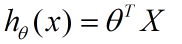其中，X为输入变量，θ为参数。
假设误差估计函数（error function）为J,我们的目标便是寻找合适的参数θ，使得误差函数J最小化。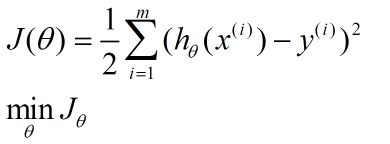调整θ以使得J(θ)取得最小值有很多方法，其中有最小二乘法(min square)，梯度下降法（gradient descent）,牛顿法（Newton’s Method）等，每种方法都有各自的优缺点。
三、 梯度下降法
在选定线性回归模型后，只需要确定参数θ，就可以将模型用来预测。然而θ需要在J(θ)最小的情况下才能确定。因此问题归结为求极小值问题，使用梯度下降法。梯度下降法最大的问题是求得有可能是全局极小值，这与初始点的选取有关。
梯度下降法步骤：
（1）初始化θ；
（2）改变θ的值，使得J(θ)按梯度下降的方向进行减少。
梯度方向由J(θ)对θ的偏导数确定，由于求的是极小值，因此梯度方向是偏导数的反方向。结果为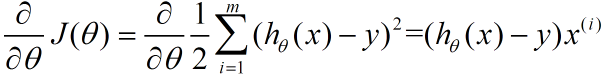所以θ的更新公式为：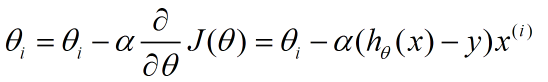其中a为步长因子，其取值要适中，太小的时收敛速度太慢，太大时有可能跳过最优解。
四、 算法实现
1. 选取参数theta0=0.75 theta1=1.65作为初始值。
2. 步长因子alpha=0.001（以上选取经试验验证有更好的收敛效果）
3. 按照梯度下降算法步骤更新theta值，设置循环次数为500次，当前j值与上一次循环得到的j值的欧式距离小于0.001时认为算法收敛，跳出循环。
4. 带入假设函数可得回归曲线。
五、 试验结果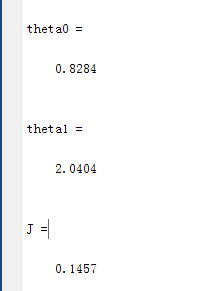theta0=0.8284
theta1=2.0404
j=0.1457
实际迭代次数4
回归曲线如图：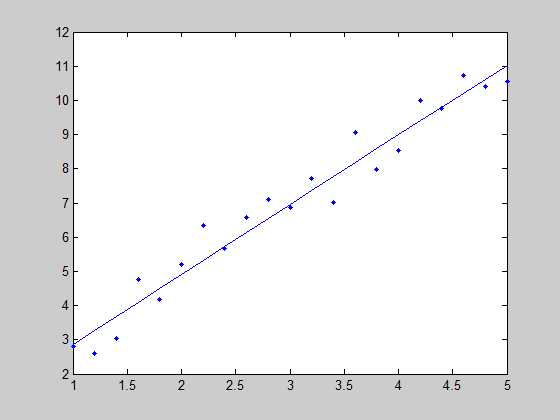六、 实验代码
clc;
clear;
load shuju.mat;
plot(X,Y,'.');
hold;
syms theta0 theta1 alpha;
theta0=0.75;
theta1=1.65;
alpha=0.001;
j=0;
for q=1:500
J=j;
j=0;
for i=1:21
j=j+0.5*(theta0+theta1*X(i)-Y(i))^2;
end
j=j/21;
if sqrt((j-J)^2)<0.001
break;
end
theta0=theta0-alpha*0.5*(theta0*theta1*X(i)-Y(i));
theta1=theta1-alpha*0.5*(theta0*theta1*X(i)-Y(i))*X(i);
end
y=theta0+theta1*X;
plot(X,y)
j
theta0
theta1
J


展开全文算法 迭代 文档
• 请按要求完成实验。建议使用 python 编程实现. 二、实验的硬件、软件平台 硬 件： 计算机 软 件： 操作系统：Linux / windows 应用软件： pycharm 三、实验内容及步骤 1）请导入数据并进行数据可视化，观察数据分布...逻辑回归
• 课程名称: 机器学习 课程类型: 选修 实验题目: 多项式拟合正弦函数 学号: 1170300511 姓名: 易亚玲 一、实验目的 掌握最小二乘法求解（无惩罚项的损失函数） 掌握加惩罚项（2范数）的损失函数优化 掌握...
• 里面的代码是用matlab实现的。matlab版本是R2018b。代码都是自己运行过的，是可以使用的。里面包括了我的实验报告，每份报告分数基本都在88-92分，包括了实验流程和心得体会，基本也可以满足要求
• 决策树前言一、实验目的二、实验内容1、导入数据2、划分训练集与测试集3、多元...而且机器学习部分的实验内容多数要求调用matlab自带函数，因此也没有什么可讲的变成部分。下面的部分，我直接使用了实验报告的内容。 一可视化 算法
• 机器学习实验三------神经网络模型实现 一、实验目的 理解神经网络相关原理和概念； 能够使用神经网络解决相关问题； 熟练使用Scikit-learn 中神经网络相关模块。 二、实验内容 使用神经网络解决糖尿病病情预测问题...神经网络 深度学习 python
• 课程名称: 机器学习 课程类型: 选修 实验题目: 多项式拟合正弦函数 学号: 姓名: 一、实验目的 理解逻辑回归模型 掌握逻辑...
• 实验要求 对问题进行简单描述并给出线性判别分析和对数几率回归解决分类问题的原理； 将问题相关数据集划分成训练集和测试集； 给出线性判别分析和对数几率回归解决手写数字识别问题的代码； 对训练后的模型进行...
• 一、 参数说明 1、在开始贝叶斯判定前，先要设置...先验概率在“sample.txt”文件中进行设置，具体sample.txt文件格式要求如下： 2、样例文件格式说明 文件开头是各个类的先验概率，有多少个类就应该有多少个先验概...
• 随机产生10000组正样本和20000负样本高斯分布的数据集合（维数设为二维），要求正样本：均值为[1;3]，方差为[20;02]；负样本：均值为[10;20]，方差为[100;010]。先验概率按样本量设定为1/3和2/3.分别利用最小错误...贝叶斯
• 《统计学习方法》第二章感知机 感知机算法详解 主要理解其损失函数，以及对其求导，这样就能迭代出更新权重www和常数bbb。 要求：生成四个高斯分布的数据，逻辑与的二分类形式。即（1,1）为正分类，（0,0），（0,1）...
• 机器学习算法模型搭建实验（人口收入普查分析）机器学习算法模型搭建实验（人口收入普查分析）具体步骤如下：1.下载数据源2.清洗数据3.构造机器学习平台模型1.登录腾讯云控制台，到云产品里选择智能钛机器学习平台3....人工智能 数据分析
• 计算学部《机器学习》课程 Lab2 实验报告 姓名 学号 班号 电子邮件 手机号码 1 实验目的 理解逻辑回归模型，掌握逻辑回归模型的参数估计算法。 2 实验...逻辑回归
• Apriori算法一、实验目的二、基本要求三、实验软件四、实验内容五、实验过程六、实验结果与评估七、实验代码 一、实验目的 本实验课程是计算机、智能、物联网等专业学生的一门专业课程...1.实验前，复习《机器学习与数数据挖掘 数据库 算法 python 关联分析
• 图像分类一、实验目的二、基本要求三、实验软件四、实验内容五、实验过程与实现六、实验结果与评估七、实验代码 一、实验目的 本实验课程是计算机、智能、物联网等专业学生的一门...1.实验前，复习《机器学习与数据挖数据挖掘 算法 python 人工智能
• 决策树算法一、实验目的二、基本要求三、实验软件四、实验内容五、实验过程六、实验结果与评估七、实验代码 一、实验目的 本实验课程是计算机、智能、物联网等专业学生的一门...1.实验前，复习《机器学习与数据挖掘》决策树 可视化 数据挖掘 python 算法
• 一、实验目的 本实验课程是计算机、智能、物联网等专业学生的一门专业课程，...1.实验前，复习《机器学习与数据挖掘》课程中的有关内容。 2.准备好实验数据。 3.编程要独立完成，程序应加适当的注释。 4.完成实验报告。数据挖掘 python 算法 深度学习
• 计算学部《机器学习》课程 Lab3 实验报告 姓名 学号 班号 电子邮件 手机号码 1 实验目的 实现一个k-means算法和混合高斯模型，并且用EM算法估计模型中的参数...聚类 算法
• 二、实验要求及实验环境 实验要求 生成数据，加入噪声； 用高阶多项式函数拟合曲线； 用解析解求解两种loss的最优解（无正则项和有正则项） 优化方法求解最优解（梯度下降，共轭梯度）； 用你得到的实验...python pycharm 矩阵
• 二、实验要求及实验环境 要求： 实现两种损失函数的参数估计（1，无惩罚项；2.加入对参数的惩罚），可以采用梯度下降、共轭梯度或者牛顿法等。 实验环境： windows10、python3.7.4、pycharm2020.2 三、数学原理 3.1 ...python pycharm
• 数据科学实验四：机器学习-聚类和回归 包含原代码：完成了实验要求的各项内容，并且对每个部分具体加了注释，易于理解 改好路径，代码直接可运行
• kNN实验 手写数字识别 实验内容 使用K-NN算法识别数字0-9，实现最基本的KNN算法，使用trainingDigits文件夹下的数据，对testDigits中的数据...实验要求 1.要求给出代码，以及运行窗口截图。 2.K对正确率的影响，...手写数字识别
• 实验室课程的目的是让您学习如何将不同的概念应用于行为数据的分析以及适用于预测任务的机器学习方法的实现。 通过这些教程，我们旨在为您提供有关如何设置编程环境以及如何以及如何使用最重要的程序包来处理行为...
• 实验目的及要求 实验源于“2019大数据挑战赛-预选赛”。 本预选赛要求选手建立文本情感分类模型，选手用训练好的模型对测试集中的文本情感进行预测，判断其情感为「Negative」或者「Positive」。所提交的结果按照...文本情感分类 二分类 python
• 基本要求：在统计学习、深度学习、强化学习、推荐系统、跨模态检索、多媒体分析等相关方向有两年以上研究项目经验，且有CCF B以上学术论文或相关大赛获奖。加分条件：国内外AI名校博士在读，目标为转正或长期实习，...
• 实验要求：给出数据预处理过程、关联规则发现算法及发现关联规则，并对关联规则结果进行分析说明。 实验题目：蔬菜价格相关性分析 蔬菜的价格会受季节、天气等多方面因素的影响，但许多会出现同涨或者同跌等现象，请......